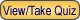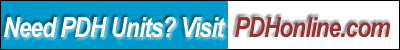Print this page

Electrical Fundamentals – Capacitors and Capacitance

A. Bhatia, B.E.

Course Outline

Capacitance is the property that characterizes its ability to store an electric charge between two conducting bodies. An electronic device called a capacitor is designed to provide capacitance in an electric circuit by providing a means for storing energy in an electric field between two conducting plates. Larger the plates, the more charges will exist and the closer the plates, the stronger will be the electrical attraction between the plates.

This 4-hr course provides a basic overview of capacitance theory and is based entirely on Naval Education and Training Materials (NAVEDTRA 14174), Electricity and Electronic Training Series; Module-2, Chapter 3, titled “Capacitance”.

The course includes a multiple-choice quiz at the end, which is designed to enhance the understanding of course materials.

Learning Objective

At the conclusion of this course, the student will be able to:

• Define the terms capacitance and state three factors that affect the value of capacitance;
• State four characteristics of electrostatic lines of force;
• Solve the capacitance from given data of the dielectric constant, the area of plates and the distance between them;
• State the difference between different types of capacitors;
• State two types of power losses associated with capacitors;
• Define the term "working voltage" of a capacitor, and compute the working voltage of a capacitor;
• State what happens to the electrons in a capacitor when the capacitor is charging and when it is discharging;
• State the relationship between voltage and time in an RC circuit when the circuit is charging and discharging;
• Calculate the value of total capacitance in a circuit containing capacitors of known value in series and parallel circuits;
• State the difference between different types of capacitors;
• State the relationship between the voltage drop across a resistor and the source voltage in an RC circuit; and
• Calculate the value of total capacitance in a circuit containing capacitors of known value in series and parallel.

Intended Audience

This course is aimed at students, professional engineers, service technicians, energy auditors, operational & maintenance personnel, facility engineers and general audience.

Course Introduction

A capacitor in its simplest form consists of two conducting plates separated by an insulating layer called a dielectric. When a capacitor is connected in a circuit across a voltage source, the voltage forces electrons onto the surface of one plate and pulls electrons off the surface of the other plate resulting in a potential difference between the plates. Because the dielectric between the plates is an insulator, current cannot flow through it. A capacitor has a finite amount of capacity to store charges and when a capacitor reaches its capacity, it is fully charged.

Capacitance is measured in farads, which is named after Michael Faraday (1791-1867). The symbol for farads is F. If a charge of 1 coulomb is placed on the plates of a capacitor and the potential difference between them is 1 volt, the capacitance is then defined to be 1 farad. One coulomb is equal to the charge of 6.25 x 1018 electrons. One farad is an extremely large quantity of capacitance. Microfarads (10-6 F) and picofarads (10-12 F) are more commonly used.

This course explains the basic concepts of capacitance and is followed by multiple – choice quiz at the end.

Course Content

In this course, you are required to study Naval Education and Training Materials (NAVEDTRA 14173), Electricity and Electronic Training Series; Module-2, 3, titled “Capacitance”:

Capacitance (Chapter 3, NAVEDTRA 14173)

Please click on the above underlined hypertexts to view, download or print the documents for your study. Because of the large file size, we recommend that you first save the file to your computer by right clicking the mouse and choosing "Save Target As ...", and then open the file in Adobe Acrobat Reader.

Course Summary

Capacitance is a measure of the amount of electric charge stored (or separated) for a given electric potential. The most common form of charge storage device is a two-plate capacitor. The capacitance of a capacitor is proportional to the quantity of charge that can be stored in it for each volt difference in potential between its plates. Mathematically this relationship is written as:

C = Q/V

Where C is capacitance in farads, Q is the quantity of stored electrical charge in coulombs, and V is the difference in potential in volts.
Therefore, stored electric charge can be calculated using the formula:

Q = CV

The difference in potential or voltage of the capacitor can be calculated using the formula:

V = Q/C

The capacitance of a capacitor is affected by three factors:

1. The area of the plates
2. The distance between the plates
3. The dielectric constant of the material between the plates

Capacitance is directly proportional to the electrostatic force field between the plates. This field is stronger when the plates are closer together. Therefore, as the distance between the plates decreases, capacitance increases. Also capacitance is directly proportional to the plate area. Larger plates provide greater capacity to store electric charge. Therefore, as the area of the plates increase, capacitance increases.

Capacitors are charged and discharged as needed by its application. Capacitors differ in size and arrangements of plates and the type of dielectric materials used. Paper, ceramic, air, mica, and electrolytic materials can be used, depending on the type of dielectric needed.

Quiz

Once you finish studying the above course content, you need to take a quiz to obtain the PDH credits.DISCLAIMER: The materials contained in the online course are not intended as a representation or warranty on the part of PDH Center or any other person/organization named herein. The materials are for general information only. They are not a substitute for competent professional advice. Application of this information to a specific project should be reviewed by a registered architect and/or professional engineer/surveyor. Anyone making use of the information set forth herein does so at their own risk and assumes any and all resulting liability arising therefrom.